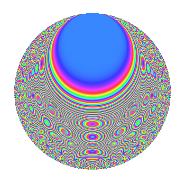# Properties

 Label 1350.2.mLevel 1350 Weight 2 Character orbit m Rep. character $$\chi_{1350}(109,\cdot)$$ Character field $$\Q(\zeta_{10})$$ Dimension 160 Sturm bound 540

# Learn more about

## Defining parameters

 Level: $$N$$ = $$1350 = 2 \cdot 3^{3} \cdot 5^{2}$$ Weight: $$k$$ = $$2$$ Character orbit: $$[\chi]$$ = 1350.m (of order $$10$$ and degree $$4$$) Character conductor: $$\operatorname{cond}(\chi)$$ = $$25$$ Character field: $$\Q(\zeta_{10})$$ Sturm bound: $$540$$

## Dimensions

The following table gives the dimensions of various subspaces of $$M_{2}(1350, [\chi])$$.

Total New Old
Modular forms 1128 160 968
Cusp forms 1032 160 872
Eisenstein series 96 0 96

## Trace form

 $$160q$$ $$\mathstrut +\mathstrut 40q^{4}$$ $$\mathstrut +\mathstrut O(q^{10})$$ $$160q$$ $$\mathstrut +\mathstrut 40q^{4}$$ $$\mathstrut -\mathstrut 10q^{10}$$ $$\mathstrut -\mathstrut 40q^{16}$$ $$\mathstrut +\mathstrut 12q^{19}$$ $$\mathstrut +\mathstrut 20q^{22}$$ $$\mathstrut -\mathstrut 26q^{25}$$ $$\mathstrut +\mathstrut 10q^{28}$$ $$\mathstrut -\mathstrut 6q^{31}$$ $$\mathstrut -\mathstrut 24q^{34}$$ $$\mathstrut +\mathstrut 40q^{37}$$ $$\mathstrut +\mathstrut 10q^{40}$$ $$\mathstrut -\mathstrut 12q^{46}$$ $$\mathstrut -\mathstrut 100q^{49}$$ $$\mathstrut -\mathstrut 58q^{55}$$ $$\mathstrut -\mathstrut 40q^{61}$$ $$\mathstrut +\mathstrut 40q^{64}$$ $$\mathstrut +\mathstrut 40q^{67}$$ $$\mathstrut +\mathstrut 98q^{70}$$ $$\mathstrut +\mathstrut 8q^{76}$$ $$\mathstrut +\mathstrut 56q^{79}$$ $$\mathstrut +\mathstrut 144q^{85}$$ $$\mathstrut -\mathstrut 10q^{88}$$ $$\mathstrut -\mathstrut 20q^{91}$$ $$\mathstrut -\mathstrut 16q^{94}$$ $$\mathstrut +\mathstrut 10q^{97}$$ $$\mathstrut +\mathstrut O(q^{100})$$

## Decomposition of $$S_{2}^{\mathrm{new}}(1350, [\chi])$$ into irreducible Hecke orbits

The newforms in this space have not yet been added to the LMFDB.

## Decomposition of $$S_{2}^{\mathrm{old}}(1350, [\chi])$$ into lower level spaces

$$S_{2}^{\mathrm{old}}(1350, [\chi]) \cong$$ $$S_{2}^{\mathrm{new}}(25, [\chi])$$$$^{\oplus 8}$$$$\oplus$$$$S_{2}^{\mathrm{new}}(50, [\chi])$$$$^{\oplus 4}$$$$\oplus$$$$S_{2}^{\mathrm{new}}(75, [\chi])$$$$^{\oplus 6}$$$$\oplus$$$$S_{2}^{\mathrm{new}}(150, [\chi])$$$$^{\oplus 3}$$$$\oplus$$$$S_{2}^{\mathrm{new}}(225, [\chi])$$$$^{\oplus 4}$$$$\oplus$$$$S_{2}^{\mathrm{new}}(450, [\chi])$$$$^{\oplus 2}$$$$\oplus$$$$S_{2}^{\mathrm{new}}(675, [\chi])$$$$^{\oplus 2}$$